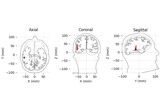# mne.DipoleFixed#

class mne.DipoleFixed(info, data, times, nave, aspect_kind, comment='', *, verbose=None)[source]#

Dipole class for fixed-position dipole fits.

Note

This class should usually not be instantiated directly via `mne.DipoleFixed(...)`. Instead, use one of the functions listed in the See Also section below.

Parameters:
info`mne.Info`

The `mne.Info` object with information about the sensors and methods of measurement.

data`array`, shape (n_channels, n_times)

The dipole data.

times`array`, shape (n_times,)

The time points.

nave`int`

Number of averages.

aspect_kind`int`

The kind of data.

comment`str`

The dipole comment.

verbose

Control verbosity of the logging output. If `None`, use the default verbosity level. See the logging documentation and `mne.verbose()` for details. Should only be passed as a keyword argument.

Notes

This class is for fixed-position dipole fits, where the position (and maybe orientation) is static over time. For sequential dipole fits, where the position can change a function of time, use `mne.Dipole`.

New in version 0.12.

Attributes:
`ch_names`

Channel names.

`times`

Time vector in seconds.

`tmax`

Last time point.

`tmin`

First time point.

Methods

 Copy the DipoleFixed object. `crop`([tmin, tmax, include_tmax, verbose]) Crop data to a given time interval. `decimate`(decim[, offset, verbose]) Decimate the time-series data. `plot`([show, time_unit]) Plot dipole data. `save`(fname[, verbose]) Save dipole in a .fif file. `shift_time`(tshift[, relative]) Shift time scale in epoched or evoked data. `time_as_index`(times[, use_rounding]) Convert time to indices.
property ch_names#

Channel names.

copy()[source]#

Copy the DipoleFixed object.

Returns:
instinstance of `DipoleFixed`

The copy.

Notes

New in version 0.16.

crop(tmin=None, tmax=None, include_tmax=True, verbose=None)[source]#

Crop data to a given time interval.

Parameters:
tmin

Start time of selection in seconds.

tmax

End time of selection in seconds.

include_tmax`bool`

If True (default), include tmax. If False, exclude tmax (similar to how Python indexing typically works).

New in version 0.19.

verbose

Control verbosity of the logging output. If `None`, use the default verbosity level. See the logging documentation and `mne.verbose()` for details. Should only be passed as a keyword argument.

Returns:
inst

The cropped time-series object, modified in-place.

Notes

Unlike Python slices, MNE time intervals by default include both their end points; `crop(tmin, tmax)` returns the interval `tmin <= t <= tmax`. Pass `include_tmax=False` to specify the half-open interval `tmin <= t < tmax` instead.

decimate(decim, offset=0, verbose=None)[source]#

Decimate the time-series data.

Parameters:
decim`int`

Factor by which to subsample the data.

Warning

Low-pass filtering is not performed, this simply selects every Nth sample (where N is the value passed to `decim`), i.e., it compresses the signal (see Notes). If the data are not properly filtered, aliasing artifacts may occur.

offset`int`

Apply an offset to where the decimation starts relative to the sample corresponding to t=0. The offset is in samples at the current sampling rate.

New in version 0.12.

verbose

Control verbosity of the logging output. If `None`, use the default verbosity level. See the logging documentation and `mne.verbose()` for details. Should only be passed as a keyword argument.

Returns:
instMNE-object

The decimated object.

Notes

For historical reasons, `decim` / “decimation” refers to simply subselecting samples from a given signal. This contrasts with the broader signal processing literature, where decimation is defined as (quoting , p. 172; which cites ):

“… a general system for downsampling by a factor of M is the one shown in Figure 4.23. Such a system is called a decimator, and downsampling by lowpass filtering followed by compression [i.e, subselecting samples] has been termed decimation (Crochiere and Rabiner, 1983).”

Hence “decimation” in MNE is what is considered “compression” in the signal processing community.

Decimation can be done multiple times. For example, `inst.decimate(2).decimate(2)` will be the same as `inst.decimate(4)`.

If `decim` is 1, this method does not copy the underlying data.

New in version 0.10.0.

References

plot(show=True, time_unit='s')[source]#

Plot dipole data.

Parameters:
show`bool`

Call pyplot.show() at the end or not.

time_unit`str`

The units for the time axis, can be “ms” or “s” (default).

New in version 0.16.

Returns:
figinstance of `matplotlib.figure.Figure`

The figure containing the time courses.

Examples using `plot`:Source localization with equivalent current dipole (ECD) fit

Source localization with equivalent current dipole (ECD) fit
save(fname, verbose=None)[source]#

Save dipole in a .fif file.

Parameters:
fnamepath-like

The name of the .fif file. Must end with `'.fif'` or `'.fif.gz'` to make it explicit that the file contains dipole information in FIF format.

verbose

Control verbosity of the logging output. If `None`, use the default verbosity level. See the logging documentation and `mne.verbose()` for details. Should only be passed as a keyword argument.

shift_time(tshift, relative=True)[source]#

Shift time scale in epoched or evoked data.

Parameters:
tshift`float`

The (absolute or relative) time shift in seconds. If `relative` is True, positive tshift increases the time value associated with each sample, while negative tshift decreases it.

relative`bool`

If True, increase or decrease time values by `tshift` seconds. Otherwise, shift the time values such that the time of the first sample equals `tshift`.

Returns:
epochsMNE-object

The modified instance.

Notes

This method allows you to shift the time values associated with each data sample by an arbitrary amount. It does not resample the signal or change the data values in any way.

time_as_index(times, use_rounding=False)[source]#

Convert time to indices.

Parameters:
timeslist-like | `float` | `int`

List of numbers or a number representing points in time.

use_rounding`bool`

If True, use rounding (instead of truncation) when converting times to indices. This can help avoid non-unique indices.

Returns:
index`ndarray`

Indices corresponding to the times supplied.

property times#

Time vector in seconds.

property tmax#

Last time point.

property tmin#

First time point.

## Examples using `mne.DipoleFixed`#Source localization with equivalent current dipole (ECD) fit

Source localization with equivalent current dipole (ECD) fit